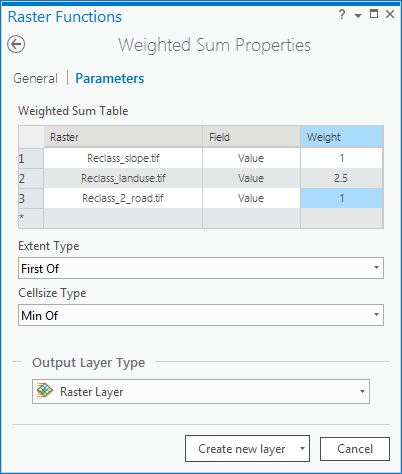# Weighted Sum function

## Overview

The Weighted Sum function allows you to overlay several rasters, multiplying each by their given weight and summing them together.## Notes

Input rasters can be integer or floating point.

The weight will be applied to the specified field for the input raster. Fields can be of type short or long integer, double, or float.

A useful way to add several rasters together is to input multiple rasters and set all weights equal to 1.

The weight values can be any positive or negative decimal value. It is not restricted to a relative percentage, nor does it need to be equal to 1.0.

## Parameters

Parameter nameDescription

Weighted Sum Table

The weighted sum table is defined by three properties:

• Raster—The raster being weighted.
• Field—The field of the raster to use for weighting.
• Weight—The weight value by which to multiply the raster. It can be any positive or negative decimal value.

Cellsize Type

Choose which cell size to use in the output raster. If all the input cell sizes are the same, all the options will yield the same results.

• First Of—Use the first cell size of the input rasters.
• Min Of—Use the smallest cell size of all the input rasters.
• Max Of—Use the largest cell size of all the input rasters. This is the default.
• Mean Of—Use the mean cell size of all the input rasters.
• Last Of—Use the last cell size of the input rasters.

Extent Type

Choose which extent should be used in the output raster:

• First Of—Use the extent of the first input raster to determine the processing extent.

• Intersection Of—Use the extent of the overlapping pixels to determine the processing extent. This is the default.
• Union Of—Use the extent of all the rasters to determine the processing extent.
• Last Of—Use the extent of the last input raster to determine the processing extent.

The Weighted Sum function provides the ability to weight and combine multiple inputs to create an integrated analysis. It is similar to the Weighted Overlay function in that multiple raster inputs, representing multiple factors, can be easily combined, incorporating weights or relative importance.

Weighted Sum works by multiplying the designated field values for each input raster by the specified weight. It then sums (adds) all input rasters together to create an output raster.

There are two major differences between these functions:

• The Weighted Sum function does not rescale the reclassified values back to an evaluation scale.
• The Weighted Sum function allows floating-point and integer values, whereas the Weighted Overlay function only accepts integer rasters as inputs.

By not rescaling the reclassified values back to the evaluation scale, the analysis maintains its resolution. For example, in a suitability model, if there are 10 input criteria that were reclassified to a scale of 1 to 10 (10 being the most favorable) and no weights were assigned, the values on the output from Weighted Sum could range from 10 to 100. For the same input, Weighted Overlay would normalize the 10-to-100 reclassified analysis range to the evaluation scale, for example, back to the 1-to-10 scale. Maintaining the model resolution in Weighted Sum can be useful when you want to identify only the top few favorable locations or a specified number of sites.

##### Note:

Combining multiple continuous data layers with different ranges is not always meaningful.

Generally, the values of continuous rasters are grouped into categories. For example, the various slope values can be grouped into flat, moderate, steep, and very steep. Each slope value can be assigned to one of these categories, and the category can be assigned a reclass value identifying the preference for the class relative to a criterion in the overlay analysis. The Reclassify tool allows such rasters to be reclassified.

The Weighted Overlay tool is used most commonly for suitability modeling and should be used to ensure correct methodologies are followed. The Weighted Sum tool is useful when you want to maintain the model resolution or when floating-point output or decimal weights are required.